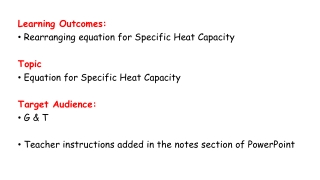DownloadDownload PresentationLearning Outcomes: Rearranging equation for Specific Heat Capacity Topic

# Learning Outcomes: Rearranging equation for Specific Heat Capacity Topic

Download Presentation## Learning Outcomes: Rearranging equation for Specific Heat Capacity Topic

- - - - - - - - - - - - - - - - - - - - - - - - - - - E N D - - - - - - - - - - - - - - - - - - - - - - - - - - -
##### Presentation Transcript

1. Learning Outcomes: • Rearranging equation for Specific Heat Capacity Topic • Equation for Specific Heat Capacity Target Audience: • G & T • Teacher instructions added in the notes section of PowerPoint

2. Starter: Which factors would affect the temperature rise of a metal block when it is heated?

3. Investigation – heating aluminium and copper Thermometer Thermometer Heater Heater Aluminium Copper

4. Identify variables for the investigation • Independent • Dependent • Control • Material (Specific Heat Capacity) • Temperature Change (θ) • Mass (1kg) and Energy supplied (J)

5. Equation for Specific Heat Capacity • E is the energy transferred in joules, J • m is the mass of the substances in kg • c is the specific heat capacity in J / kg °C • θ (‘theta’) is the temperature change in degrees Celsius, °C E = m × c × θ

6. What did we find out from our investigation? How can we use the formula E = m x c x θ to calculate the temperature of the aluminium and copper blocks from our results?

7. To Do… • Complete worksheet